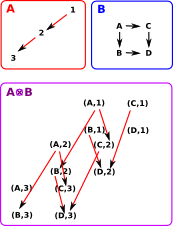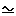# Maths - Multiplication of graph

On this page we give an example for the monoidal categories page. This is especially to illustrate cartesian and tensor products. We could do something similar in categories, although in categories we have the composition of all arrows and the diagrams would be a lot more cluttered, so I have not done that here. However we could think of these graphs as generators for categories.

## Cartesian Product

We can extend the concept of cartesian product of sets to categories as follows:

 Here is an ordinary cartesian product of sets.Here we have sets with structure, represented by arrows. An arrow (a, b) -> (a', b') exists in A×B if and only if either: a = a' and b->b' exists in A, or b = b' and a->a' exists in B.## Tensor Product

We can extend the concept of cartesian product of sets to categories as follows:

 The objects are the same as cartesian product of sets but the arrows are different from the cartesian example above. There is an arrow in AB only if there is an arrow in both A and B. An arrow (a, b) -> (a', b') exists in AB if and only if: b->b' exists. and a->a' exists.Looking at the case above it looks like we could recover projections to A and B from AB. However, is there an example where we can't, where one of the operands has a object with no arrows leaving it? On the right is a diagram of an extreme case where one of the operands has no arrows. In this case, by the definition given above, the tensor product will have no arrows. In this case there may be a projection from A0 to 0 (that would be the Cartesian set product) Would there be an arrow from A0 to A? maybe so, if we take a graph with no arrows as being an initial object. Perhaps this is an example which shows the cartesian product of graphs is not a product in the category theory sense?A graph with a single object and an arrow to itself is the unit: 1.## Exponential Object

 There is a homset that shows all the ways an object can be mapped into another object:This is related to the Cartesian product by Currying: Hom(A×B,C)Hom(A,CB) The related product might look like this: